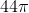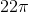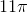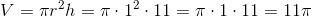Example Questions

← Previous 1

Example Question #1 : How To Find The Volume Of A Cylinder

A certain cylinder has diameter that is twice the length of its height. If the volume of the cylinder is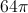cubic inches, what is its radius?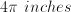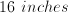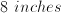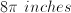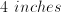Explanation:

The volume of a cylinder is: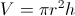You can think of the volume as the area of the base times the height. Since it is given that the diameter is twice the length of the height, the radius (half the diameter) equals the height. If it helps to visualize these dimensions, draw the cylinder described.

The equation can be rewritten, using the height in terms of the radius.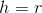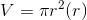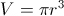Plug in the given volume to solve for the radius.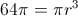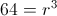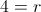Example Question #1 : How To Find The Volume Of A Cylinder

The radius of a cylinder is five and its height is nine. What is its volume?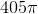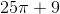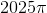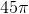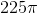Explanation:

To solve this question, you must remember that the formula for volume is the product of the area of the base and the height. The area of the base of this cylinder is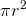.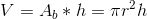Plug in the given radius and height to solve.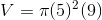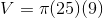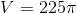Example Question #3 : Cylinders

What is the volume of a round metal washer with an outer radius of 8 in, an inner radius of 2 in, and a thickness of 0.5 in?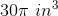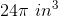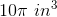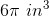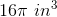Explanation:

The volume of a cylinder is given by the formula: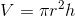.

For a shape with a hole through the center, the final volume is equal to the total volume of the shape minus the volume of the inner hole. In this question, we are looking for the volume given by the larger radius minus the volume given by the smaller radius. The height is equal to the thickness of the washer.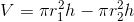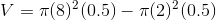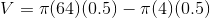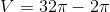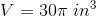Example Question #4 : Cylinders

How much more volume can a cylinder hold than a cone given that both have the same radius and height?

Here B represents the area of the Base, and h the height.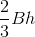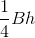None of the answers are correct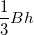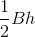Explanation:

Cylinder: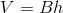Cone: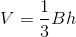Thus the difference is 2/3Bh and that means a cylinder can hold 2/3Bh more given the same radius and height.

Example Question #5 : Cylinders

The height of a right circular cylinder is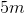and its radius is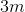. What is the volume, in cubic meters, of the cylinder?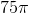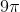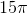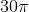Explanation:

The volume of a right circular cylinder is equal to its height () multiplied by the area of the circle base ().

In this scenario, the Volume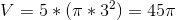.

Therefore, the volume is.

Example Question #1 : How To Find The Volume Of A Cylinder

What is the volume of a cylinder with a radius of four inches and a height of seven inches?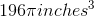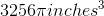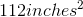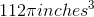Explanation:

Plug the radius and height into the formula for the volume of a cylinder: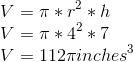Example Question #7 : Cylinders

What is the volume of a cylinder with a base diameter of 12 and a height of 3? Leave your answer in terms of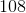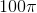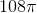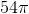Explanation:

To find the volume of a cylinder use formula: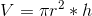For a cylinder with a radius of 6 and a height of 3 this yields: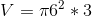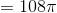Example Question #2 : How To Find The Volume Of A Cylinder

Find the volume of a cylinder whose diameter isand height is.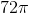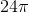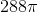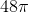Explanation:

To find volume, simply use the following formula. Remember, you were given diameter so radius is half of that. Thus,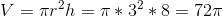Example Question #1 : How To Find The Volume Of A Cylinder

Find the volume of cylinder with diameter ofand height of.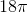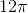Explanation:

To find volume of a cylinder, simply use the following formula. Thus,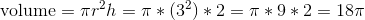Example Question #1 : How To Find The Volume Of A Cylinder

Find the volume fo a cylinder whose radius isand height is.